Open in App
Not now

# Bernoulli’s Principle

• Last Updated : 11 Nov, 2022

Bernoulli’s Principle is an important formula used in Fluid Mechanics. Bernoulli principle is also called by the term Bernoulli’s Equation or Bernoulli Theorem. Bernoulli’s Principle provides the relationship between the pressure (P) of the fluid flowing, at a height (h) of the container having kinetic and gravitational potential energy. This principle was first stated by Daniel Bernoulli and then formulated in Bernoulli’s Equation by Leonhard Euler in 1752. The conservation of energy was found to be true for flowing fluids by the statement of Bernoulli’s Principle. It may seem contradictory, but Bernoulli’s principle describes how a fluid’s velocity and pressure are related to each other. Many people believe that Bernoulli’s principle shouldn’t hold true, yet this belief may be based on an insufficient understanding of what Bernoulli’s principle truly states.

## What is Bernoulli’s Principle?

Bernoulli’s Principle says that when a fluid is flowing horizontally, the points where the speed is higher exhibit low pressure, while the points where the speed is lower exhibit high pressure.

According to Bernoulli’s principle, the gravitational potential energy of elevation, the energy related to fluid pressure, and the kinetic energy of the fluid motion combine up to give the total mechanical energy of a flowing fluid, and are all constant.

Bernoulli’s Principle phenomenon can be observed in rivers. In some places, the width of the river was found to be changed. When the width of the river is wider the speed of the water flowing through it decreases. While the speed of water increases in the narrower regions.

Hence, the principle of conservation of energy can be used to derive Bernoulli’s Equation.

## Bernoulli’s Principle Formula

Bernoulli’s Principle is formulated into an equation called Bernoulli’s Equation. Bernoulli’s Equation is a relationship between kinetic energy, gravitational potential energy, and the pressure of the fluid inside the container.

Thus, Bernoulli’s Principle Formula is stated as:where,

• P is the Pressure exerted by the fluid,
• ρ is the Density of the fluid,
• v is the Velocity of the fluid,
• g is the Acceleration due to gravity and
• h is the Height of the container.

## Derivation of Bernoulli’s Equation

Let us consider a container in the shape of a pipe, whose two edges are placed at different heights and varying diameters. The relationship between the areas of cross-sections A, the flow speed v, height from the ground y, and pressure P at two different points 1 and 2 are given in the figure below.

As illustrated in the diagram, the pipe should be at various heights. According to the equation of continuity, Its velocity must fluctuate. To achieve this acceleration and force are required, which is produced by the fluid surrounding it, and the pressure in different places must be different.

Before, deriving Bernoulli’s Equation, we assume that the fluid has no viscous forces, so the fluid’s energy will be conserved. Also, the density of the fluid flowing at both points of the container must be uniform.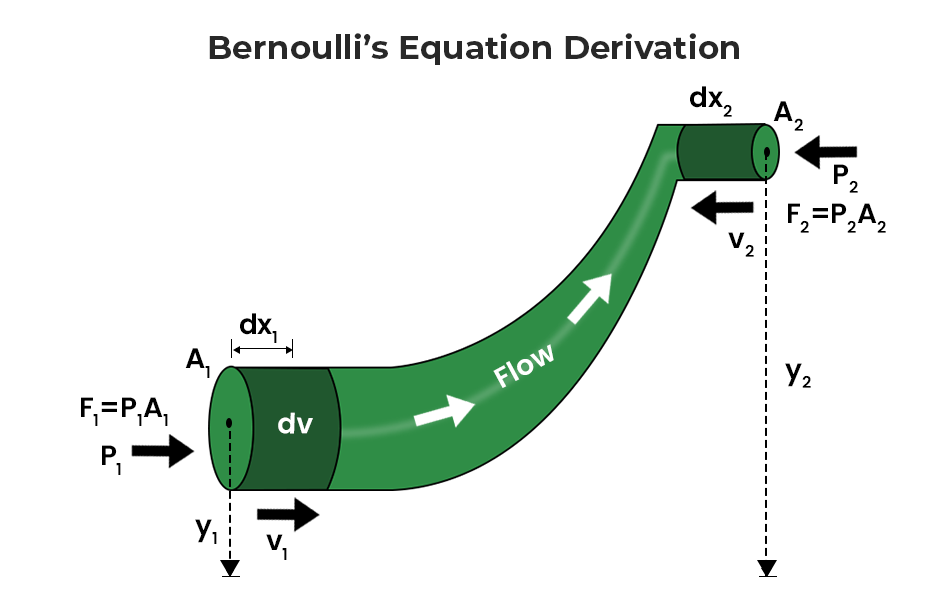To determine Bernoulli’s equation, we must first determine how much work done experienced by the fluid,

The work done at point 1 where the force F1 is exerted to displace the fluid to dx1 is

dW1 = F1 dx1

Here, the force exerted at point 1 is given by, F1 = P1A

where P1 and A1 are the pressure exerted and cross-sectional area at point 1.

This implies,

dW1 = P1A1 dx1

= P1 dv

Similarly, the work done by the fluid at point 2 is:

dW2 = P2A2 dx2

= P2 dv

Now, the total work done by the fluid flowing through the container is,

dW = P1 dv – P2 dv

= (P1 – P2) dv      ….. (1)

Now, the change in the kinetic energy of the fluid is given by,But, the mass m of the fluid can be written as,

m = ρ dv

Therefore,….. (2)

Similarly, the change in gravitational potential energy is given by,….. (3)

According to the law of conservation of energy:

dW = dK + dUThis is Bernoulli’s equation and this can be written as a general expression,## Principle of Continuity

The primary consideration when representing the flow of fluids or gases is continuity. It shows how mass conservation applies to gases and liquids. The law of conservation of mass in fluid dynamics is demonstrated using the continuity equation.

The principle of continuity is stated as, when a incompressible fluid is flowing in a streamline flow then mass passing through the system at different cross-sectional areas are equal.

The flow and behavior of fluids in a pipe are explained by the continuity equation. This formula is used to explain the flow of liquids and gases through pipes, ducts, rivers, etc.

Consider a pipe as shown above, with different cross-sectional areas A1 and A2. Let the rate of mass entering and leaving the given system be M1 and M2 respectively, with a speed of flow v, and density ρ of the fluid.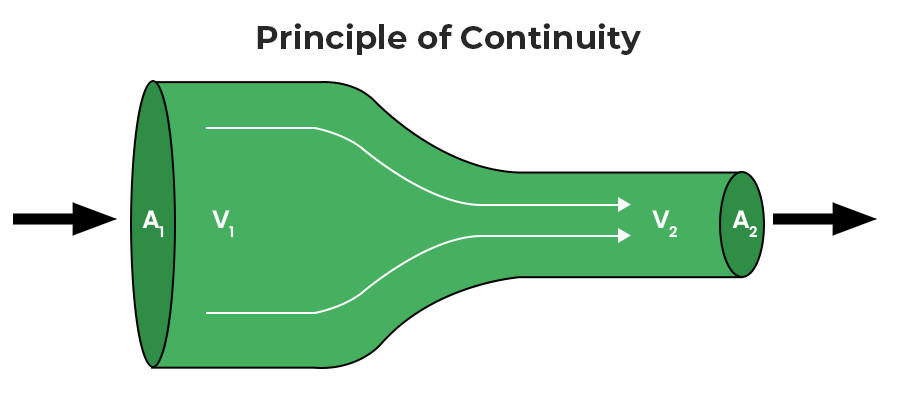Then, according to the principle of continuity, the rate of mass of fluid entering must be equal to the rate of mass of fluid leaving the system.

Since, the rate of mass entering, M1 = ρA1V1 Δt and

The rate of mass entering, M2 = ρA2V2 Δt

Therefore,

ρA1V1 Δt = ρA2V2 Δt

The above equation is now termed as Equation of Continuity.

## Application of Bernoulli’s Principle

The following are the important applications of Bernoulli’s Principle:

### Venturimeter

A venturimeter is a device used to measure the rate of flow in a pipe with a non-uniform cross-section.

To the wide and narrow sections of the pipe, we apply Bernoulli’s equation, where h1=h2. The pressure P2 is less than P1 because A1 is bigger than A2, and v2 is greater than v1. The fluid is accelerated as it enters the tube, which is narrow, and slowed as it exits by a net force to the left.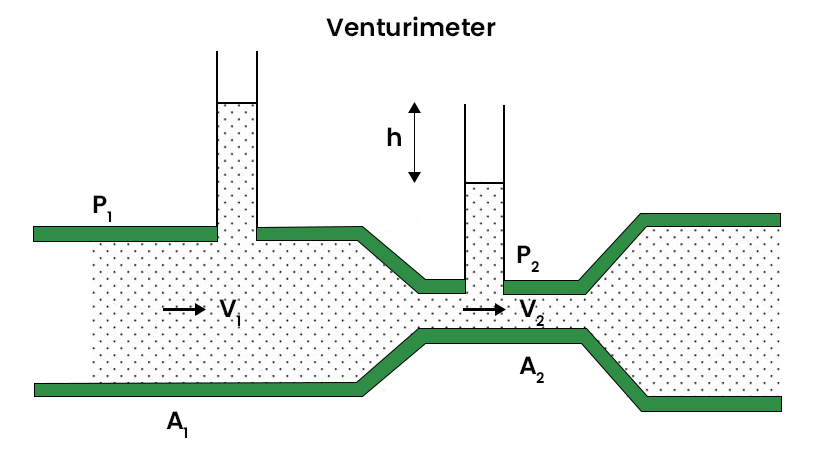The venturi meter formula is stated as,where,

• v1 is the rate of fluid flowing,
• g is the acceleration due to gravity,
• h is the difference in liquid level in the two tubes,
• A1 and A2 are the cross-sectional areas of the tube.

### Principle of Lifting an Aircraft

Bernoulli’s theorem governs the operation of airplanes. The plane’s wings have a certain form. When the plane is moving, the air flows past it at a high rate, despite the plane’s low surface wig. There is a variation in the flow of air above and below the wings due to Bernoulli’s principle. As a result of the flow of air on the wing’s up surface, this concept causes a change in pressure. If the force is greater than the plane’s mass, the plane will ascend as shown in the figure below: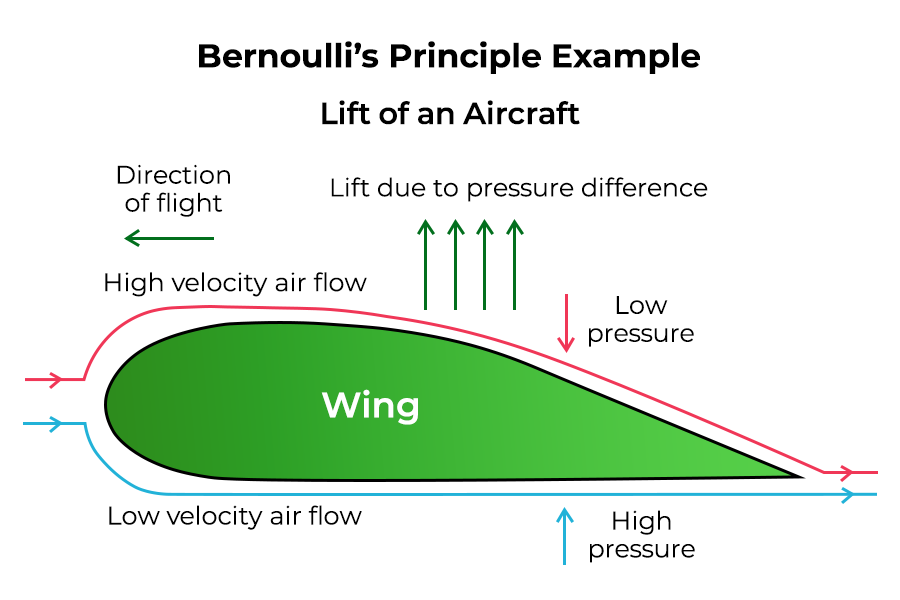### Action of Atomizer or Spray Gun

When the piston is pulled, air flows out of the horizontal tube, causing the pressure to drop which is less than the container’s pressure. The consequence is that the liquid in the vertical tube rises. When it runs into the fast air in the tube, it dissipates into a thin mist. The same idea governs filter pumps, Bunsen burners, and sprayers used to apply pesticides or scents.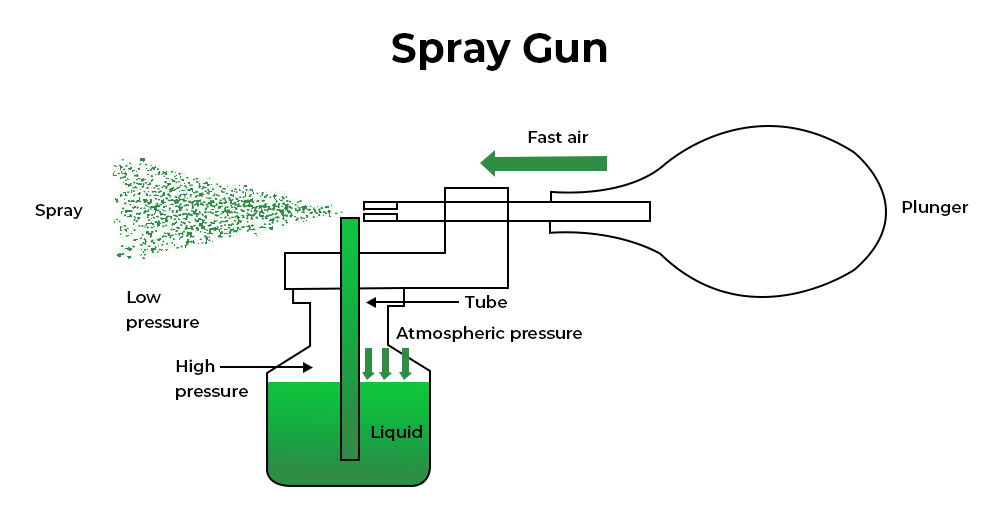## Relation between Conservation of Energy and Bernoulli’s Equation

Bernoulli’s theorem states that the total amount of these three energies within a constant moving fluid, i.e., kinetic energy, potential energy, and pressure energy, will remain steady throughout the pipeline. Regardless of these facts, one must understand several factors:

• The movement of the liquid is inferred to be constant; there is no alteration with respect to pressure, speed, and consistency of the liquid at any point in time. However, in the case of an unstable liquid flow, there will be a change in speed. Hence, this equation can be utilized and does not show an error.
• The fluid is considered incompressible. As all liquids are incompressible in nature, this principle can be applied to all fluids. However, in the case of gas flow, there should be a constant density, and one can manage with little difference in pressure, velocity, and temperature.
• Another consideration is the irrotational feature of liquids. This means that the overall angular momentum throughout the liquid is zero.
• For this principle, the type of fluid should be ideal, meaning there should be no loss of energy due to frictional activity. Hence, there is no excess energy production due to internal particle friction.

## Limitations of Bernoulli’s Principle

The following are the limitations of Bernoulli’s principle:

• Because of friction, the fluid particle velocity in the middle of a tube gradually decreases toward the tube’s direction. The velocity of the particles of the liquid is not constant, therefore the liquid’s mean velocity must be used.
• This Bernoulli equation can be used to streamline liquid supply. It is ineffective in turbulent or non-steady flow.
• The liquid flow will be affected by the liquid’s external force.
• This theorem preferably applies to non-viscosity fluids.
• Incompressible fluid is required.
• When a fluid is traveling in a curved path, the energy generated by centrifugal forces must be taken into account.
• The liquid flow should remain constant over time.
• A small amount of kinetic energy can be converted to heat energy in an unstable flow, and some energy can be lost due to shear stress in a thick flow. As a result, these losses must be overlooked.
• The effect of viscous must be negligible.

## Solved Examples on Bernoulli’s Principle

Example 1: Water is flowing at a rate of 2m3s through a tube with a diameter of 1 m. If the pressure at this point is 80 kPa, what is the pressure of the water after the tube narrows to a diameter of 0.5m? ρwater=1.0 kgl-1

Solution:

According to Bernoulli’s expression:The height is same, so the expression can be written asThe expression for the cross-sectional area isSimilarlyThe expression for the velocity for each diameter isSimilarly,Substitute the value in Bernoulli’s expression:Example 2: Explain why:

(a) The blood pressure in humans is greater at the feet than at the brain.

(b) Atmospheric pressure at a height of about 6 km decreases to nearly half of its value at sea level, though the height of the atmosphere is more than 100 km.

(c) Hydrostatic pressure is a scalar quantity even though the pressure is force divided by area.

Solution:

(a) The height of the blood column in the foot is greater than that in the brain. As a result, human blood pressure is higher in the feet than in the brain.

(b) The relationship between air density and height is not linear. As a result, pressure does not decrease linearly with height. P = P0e–αh gives the air pressure at a height h, where P0 is the pressure of air at sea level and α is a constant.

(c) When a force is applied to a liquid, the pressure is distributed evenly throughout the liquid. As a result, the pressure due to liquid has no definite direction. As a result, hydrostatic pressure is a scalar value.

Example 3: Drive the expression for Bernoulli’s principle.

Solution:

The flow of an ideal fluid in a pipe of varying cross-section. The fluid in a section of length v1∆t moves to the section of length v2∆t in time ∆t

Consider the flow at two regions BC and DE. Take a look at the flow in two different regions: BC and DE. Consider the fluid that present between B and D would travelled in an infinitesimal time interval ∆t. If v1 is the speed at B and v2 is the speed at D, the fluid at B has travelled a distance of v1∆t to C i.e.In the same interval ∆t the fluid initially at D moves to E, a distance equal to v2∆t i.e.The areas A1 and A2  has  the pressures P1 and P2. The work done on the fluid at BC iswhere  ∆V is the volume passes through region BC.

Since the same volume ∆V passes through both the regions BC and DE. therefore the expression for the work done by the fluid at the another end DE isSo the total work done on the fluid isLet the density  of the fluid is ρ therefore  the expression ∆m = ρ∆V is the  mass passing through the pipe in time ∆t, then  change in gravitational potential energy isThe change in its kinetic energy is given byAccording to he work – energy theorem’Rearrange the above expression,This is Bernoulli’s equation and this can written as general expression.

This is Bernoulli’s equation and this can written as general expression,Example 4: Suppose that a huge tank 50m high and filled with water is open to the atmosphere and is hit by a bullet that pierces one side of the tank, allowing water to flow out. The hole is 2m above the ground. If the hole is very small in comparison with the size of the tank, how quickly will the water flow out of the tank?

Solution:

According to Bernoulli’s expression:According to question it is assumed that the top of the container as point 1, and the hole where water is flowing out as point 2. Both points are open to the atmosphere. Therefore, the pressure on each side of the above equation is equal to 1 atm, and thus it got cancel. The size of the hole on the side of the tank is so small compared to the rest of the tank, the velocity of the water at point 1 is nearly equal to 0. Hence, we can cancel out theterm on the left side of the equation. The expression can rewrite as,Substitute the values in the above expression,## FAQs on Bernoulli’s Principle

Question 1: What is Bernoulli’s Equation?

Bernoulli’s Equation was first explained by Daniel Bernoulli. Bernoulli’s Principle for fluid flow is the basis of Bernoulli’s equation. The total energy of the system is always constant, as shown by Bernoulli’s equation for a fluid. It is stated in accordance with the relationship shown below.where,

• P is the Pressure exerted by the fluid,
• ρ is the Density of the fluid,
• v is the Velocity of the fluid,
• g is the Acceleration due to gravity and
• h is the Height of the container.

Question 2: Explain Head loss in Bernoulli’s equation.

The drop in total pressure, which is the sum of the velocity head, pressure head, and elevation head of the fluid moving through the hydraulic system, is represented by the head loss in Bernoulli’s equation.

Question 3: How chimney works?

In most cases, the wind speed outside the home is higher than the wind speed inside. Because of the higher wind speed above the chimney’s nozzle, the pressure drops and the air rushes from a high-pressure area to a low-pressure area, which causes the smoke to be blown away by the chimney. Thus, it is simple to see why chimneys perform better when there is a strong wind flow.

Question 4: What are some applications of Bernoulli’s Principle?